@Team 2018-06-09T13:47:31.000000Z 字数 7669 阅读 6338

# 目标检测模型的评估指标mAP详解（附代码）

叶虎

## 目标检测问题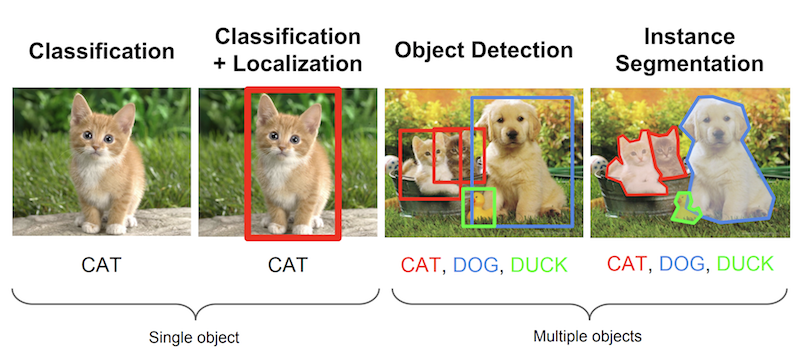## 评估目标检测模型

### 关于Ground Truth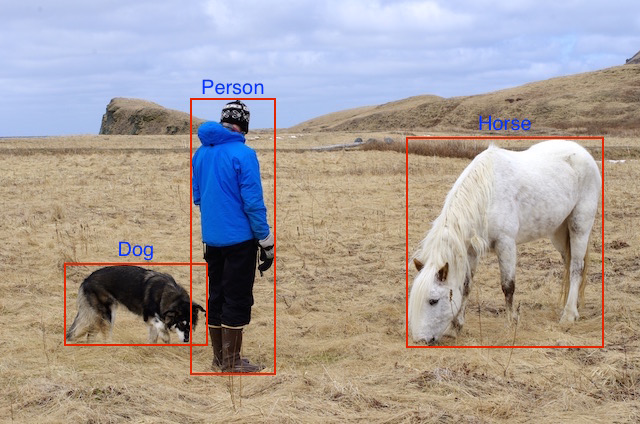Ground truth可视化## mAP含义及计算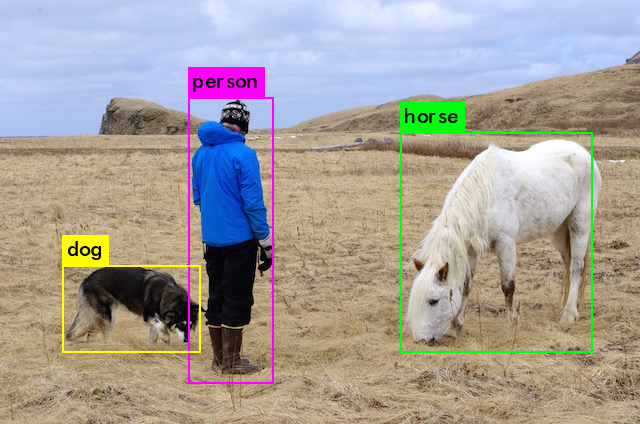### IoU

IoU是预测框与ground truth的交集和并集的比值。这个量也被称为Jaccard指数，并于20世纪初由Paul Jaccard首次提出。为了得到交集和并集，我们首先将预测框与ground truth放在一起，如图所示。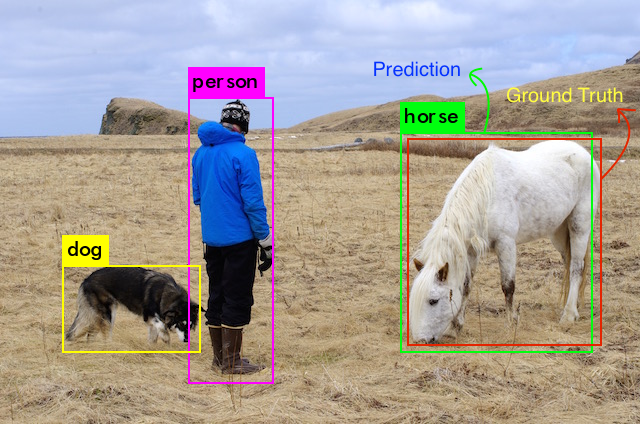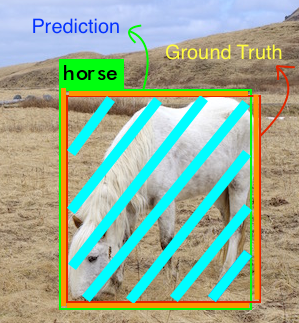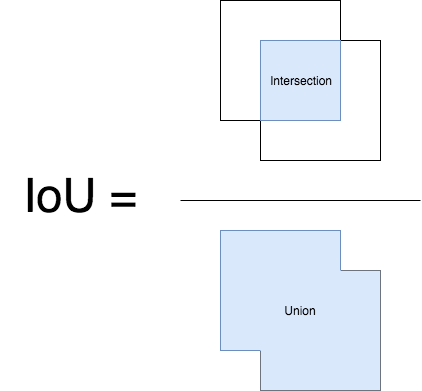### 计算mAP

mAP这个术语有不同的定义。此度量指标通常用于信息检索和目标检测领域。然而这两个领域计算mAP的方式却不相同。这里我们只谈论目标检测中的mAP计算方法。

For a given task and class, the precision/recall curve is computed from a method’s ranked output. Recall is defined as the proportion of all positive examples ranked above a given rank. Precision is the proportion of all examples above that rank which are from the positive class. The AP summarises the shape of the precision/recall curve, and is defined as the mean precision at a set of eleven equally spaced recall levels [0,0.1,...,1]: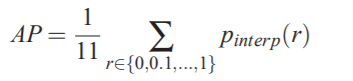The precision at each recall level r is interpolated by taking the maximum precision measured for a method for which the corresponding recall exceeds r:
The intention in interpolating the precision/recall curve in this way is to reduce the impact of the “wiggles” in the precision/recall curve, caused by small variations in the ranking of examples.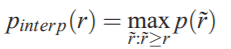Up until 2009 interpolated average precision (Salton and Mcgill 1986) was used to evaluate both classification and detection. However, from 2010 onwards the method of computing AP changed to use all data points rather than TREC-style sampling (which only sampled the monotonically decreasing curve at a fixed set of uniformly-spaced recall values 0, 0.1, 0.2,..., 1). The intention in interpolating the precision–recall curve was to reduce the impact of the ‘wiggles’ in the precision–recall curve, caused by small variations in the ranking of examples. However, the downside of this interpolation was that the evaluation was too crude to discriminate between the methods at low AP.

1. mAP通常是在一个数据集上计算得到的。
2. 虽然解释模型输出的绝对量化并不容易，但mAP作为一个相对较好的度量指标可以帮助我们。 当我们在流行的公共数据集上计算这个度量时，该度量可以很容易地用来比较目标检测问题的新旧方法。
3. 根据训练数据中各个类的分布情况，mAP值可能在某些类（具有良好的训练数据）非常高，而其他类（具有较少/不良数据）却比较低。所以你的mAP可能是中等的，但是你的模型可能对某些类非常好，对某些类非常不好。因此，建议在分析模型结果时查看各个类的AP值。这些值也许暗示你需要添加更多的训练样本。

## 代码实现

# 按照置信度降序排序
sorted_ind = np.argsort(-confidence)
BB = BB[sorted_ind, :]   # 预测框坐标
image_ids = [image_ids[x] for x in sorted_ind] # 各个预测框的对应图片id

# 便利预测框，并统计TPs和FPs
nd = len(image_ids)
tp = np.zeros(nd)
fp = np.zeros(nd)
for d in range(nd):
R = class_recs[image_ids[d]]
bb = BB[d, :].astype(float)
ovmax = -np.inf
BBGT = R['bbox'].astype(float)  # ground truth

if BBGT.size > 0:
# 计算IoU
# intersection
ixmin = np.maximum(BBGT[:, 0], bb)
iymin = np.maximum(BBGT[:, 1], bb)
ixmax = np.minimum(BBGT[:, 2], bb)
iymax = np.minimum(BBGT[:, 3], bb)
iw = np.maximum(ixmax - ixmin + 1., 0.)
ih = np.maximum(iymax - iymin + 1., 0.)
inters = iw * ih

# union
uni = ((bb - bb + 1.) * (bb - bb + 1.) +
(BBGT[:, 2] - BBGT[:, 0] + 1.) *
(BBGT[:, 3] - BBGT[:, 1] + 1.) - inters)

overlaps = inters / uni
ovmax = np.max(overlaps)
jmax = np.argmax(overlaps)
# 取最大的IoU
if ovmax > ovthresh:  # 是否大于阈值
if not R['difficult'][jmax]:  # 非difficult物体
if not R['det'][jmax]:    # 未被检测
tp[d] = 1.
R['det'][jmax] = 1    # 标记已被检测
else:
fp[d] = 1.
else:
fp[d] = 1.

# 计算precision recall
fp = np.cumsum(fp)
tp = np.cumsum(tp)
rec = tp / float(npos)
# avoid divide by zero in case the first detection matches a difficult
# ground truth
prec = tp / np.maximum(tp + fp, np.finfo(np.float64).eps)


def voc_ap(rec, prec, use_07_metric=False):
"""Compute VOC AP given precision and recall. If use_07_metric is true, uses
the VOC 07 11-point method (default:False).
"""
if use_07_metric:  # 使用07年方法
# 11 个点
ap = 0.
for t in np.arange(0., 1.1, 0.1):
if np.sum(rec >= t) == 0:
p = 0
else:
p = np.max(prec[rec >= t])  # 插值
ap = ap + p / 11.
else:  # 新方式，计算所有点
# correct AP calculation
# first append sentinel values at the end
mrec = np.concatenate(([0.], rec, [1.]))
mpre = np.concatenate(([0.], prec, [0.]))

# compute the precision 曲线值（也用了插值）
for i in range(mpre.size - 1, 0, -1):
mpre[i - 1] = np.maximum(mpre[i - 1], mpre[i])

# to calculate area under PR curve, look for points
# where X axis (recall) changes value
i = np.where(mrec[1:] != mrec[:-1])

# and sum (\Delta recall) * prec
ap = np.sum((mrec[i + 1] - mrec[i]) * mpre[i + 1])
return ap• 私有
• 公开
• 删除